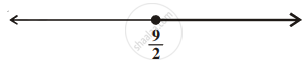# Solution of a linear inequality in variable x is represented on number line given below ______. - Mathematics

MCQ
Fill in the Blanks

Solution of a linear inequality in variable x is represented on number line given below ______.#### Options

• x ∈ (9/2, oo)

• x ∈ [9/2, oo)

• x ∈ [-oo, 9/2)

• x ∈ (-oo, 9/2]

#### Solution

Solution of a linear inequality in variable x is represented on number line underlinebb(x ∈ [9/2, oo)).
Explanation:

The given graph has all real values of x greater than and equal to 9/2.

So, x ≥ 9/2

⇒ x ∈ [9/2, oo)

Concept: Algebraic Solutions of Linear Inequalities in One Variable and Their Graphical Representation
Is there an error in this question or solution?
Chapter 6: Linear Inequalities - Exercise [Page 110]

#### APPEARS IN

NCERT Mathematics Exemplar Class 11
Chapter 6 Linear Inequalities
Exercise | Q 28 | Page 110
Share